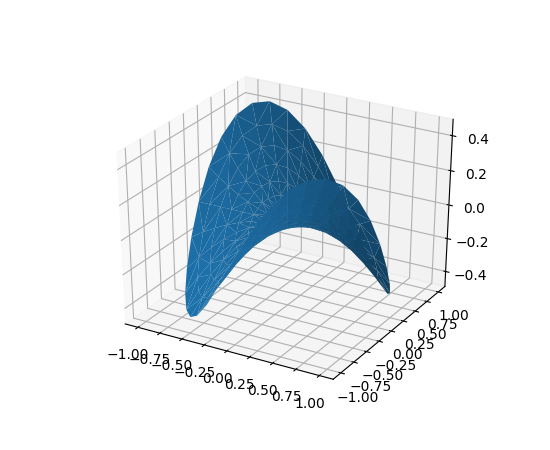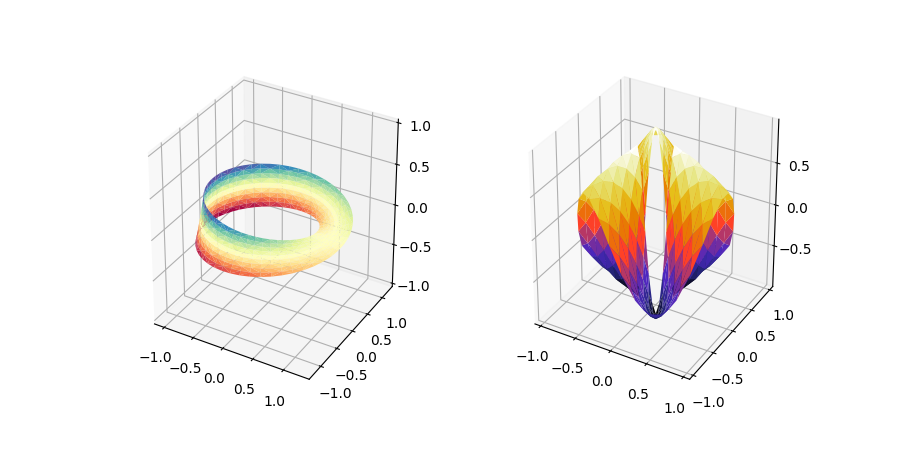# The mplot3d Toolkit¶

Generating 3D plots using the mplot3d toolkit.

## Getting started¶

An Axes3D object is created just like any other axes using the projection='3d' keyword. Create a new matplotlib.figure.Figure and add a new axes to it of type Axes3D:

import matplotlib.pyplot as plt
from mpl_toolkits.mplot3d import Axes3D
fig = plt.figure()


New in version 1.0.0: This approach is the preferred method of creating a 3D axes.

Note

Prior to version 1.0.0, the method of creating a 3D axes was different. For those using older versions of matplotlib, change ax = fig.add_subplot(111, projection='3d') to ax = Axes3D(fig).

### Line plots¶

Axes3D.plot(self, xs, ys, *args, zdir='z', **kwargs)[source]

Plot 2D or 3D data.

Parameters: xs : 1D array-like x coordinates of vertices. ys : 1D array-like y coordinates of vertices. zs : scalar or 1D array-like z coordinates of vertices; either one for all points or one for each point. zdir : {'x', 'y', 'z'} When plotting 2D data, the direction to use as z ('x', 'y' or 'z'); defaults to 'z'. **kwargs Other arguments are forwarded to matplotlib.axes.Axes.plot.

### Scatter plots¶

Axes3D.scatter(self, xs, ys, zs=0, zdir='z', s=20, c=None, depthshade=True, *args, **kwargs)[source]

Create a scatter plot.

Parameters: xs, ys : array-like The data positions. zs : float or array-like, optional, default: 0 The z-positions. Either an array of the same length as xs and ys or a single value to place all points in the same plane. zdir : {'x', 'y', 'z', '-x', '-y', '-z'}, optional, default: 'z' The axis direction for the zs. This is useful when plotting 2D data on a 3D Axes. The data must be passed as xs, ys. Setting zdir to 'y' then plots the data to the x-z-plane. See also Plot 2D data on 3D plot. s : scalar or array-like, optional, default: 20 The marker size in points**2. Either an array of the same length as xs and ys or a single value to make all markers the same size. c : color, sequence, or sequence of color, optional The marker color. Possible values: A single color format string. A sequence of color specifications of length n. A sequence of n numbers to be mapped to colors using cmap and norm. A 2-D array in which the rows are RGB or RGBA. For more details see the c argument of scatter. depthshade : bool, optional, default: True Whether to shade the scatter markers to give the appearance of depth. **kwargs All other arguments are passed on to scatter. paths : PathCollection

### Wireframe plots¶

Axes3D.plot_wireframe(self, X, Y, Z, *args, **kwargs)[source]

Plot a 3D wireframe.

Note

The rcount and ccount kwargs, which both default to 50, determine the maximum number of samples used in each direction. If the input data is larger, it will be downsampled (by slicing) to these numbers of points.

Parameters: X, Y, Z : 2d arrays Data values. rcount, ccount : int Maximum number of samples used in each direction. If the input data is larger, it will be downsampled (by slicing) to these numbers of points. Setting a count to zero causes the data to be not sampled in the corresponding direction, producing a 3D line plot rather than a wireframe plot. Defaults to 50. New in version 2.0. rstride, cstride : int Downsampling stride in each direction. These arguments are mutually exclusive with rcount and ccount. If only one of rstride or cstride is set, the other defaults to 1. Setting a stride to zero causes the data to be not sampled in the corresponding direction, producing a 3D line plot rather than a wireframe plot. 'classic' mode uses a default of rstride = cstride = 1 instead of the new default of rcount = ccount = 50. **kwargs Other arguments are forwarded to Line3DCollection.

### Surface plots¶

Axes3D.plot_surface(self, X, Y, Z, *args, norm=None, vmin=None, vmax=None, lightsource=None, **kwargs)[source]

Create a surface plot.

By default it will be colored in shades of a solid color, but it also supports color mapping by supplying the cmap argument.

Note

The rcount and ccount kwargs, which both default to 50, determine the maximum number of samples used in each direction. If the input data is larger, it will be downsampled (by slicing) to these numbers of points.

Parameters: X, Y, Z : 2d arrays Data values. rcount, ccount : int Maximum number of samples used in each direction. If the input data is larger, it will be downsampled (by slicing) to these numbers of points. Defaults to 50. New in version 2.0. rstride, cstride : int Downsampling stride in each direction. These arguments are mutually exclusive with rcount and ccount. If only one of rstride or cstride is set, the other defaults to 10. 'classic' mode uses a default of rstride = cstride = 10 instead of the new default of rcount = ccount = 50. color : color-like Color of the surface patches. cmap : Colormap Colormap of the surface patches. facecolors : array-like of colors. Colors of each individual patch. norm : Normalize Normalization for the colormap. vmin, vmax : float Bounds for the normalization. shade : bool Whether to shade the facecolors. Defaults to True. Shading is always disabled when cmap is specified. lightsource : LightSource The lightsource to use when shade is True. **kwargs Other arguments are forwarded to Poly3DCollection.

### Tri-Surface plots¶

Axes3D.plot_trisurf(self, *args, color=None, norm=None, vmin=None, vmax=None, lightsource=None, **kwargs)[source]

Plot a triangulated surface.

The (optional) triangulation can be specified in one of two ways; either:

plot_trisurf(triangulation, ...)


where triangulation is a Triangulation object, or:

plot_trisurf(X, Y, ...)
plot_trisurf(X, Y, triangles, ...)
plot_trisurf(X, Y, triangles=triangles, ...)


in which case a Triangulation object will be created. See Triangulation for a explanation of these possibilities.

The remaining arguments are:

plot_trisurf(..., Z)


where Z is the array of values to contour, one per point in the triangulation.

Parameters: X, Y, Z : array-like Data values as 1D arrays. color Color of the surface patches. cmap A colormap for the surface patches. norm : Normalize An instance of Normalize to map values to colors. vmin, vmax : scalar, optional, default: None Minimum and maximum value to map. shade : bool Whether to shade the facecolors. Defaults to True. Shading is always disabled when cmap is specified. lightsource : LightSource The lightsource to use when shade is True. **kwargs All other arguments are passed on to Poly3DCollection

ExamplesNew in version 1.2.0: This plotting function was added for the v1.2.0 release.

### Contour plots¶

Axes3D.contour(self, X, Y, Z, *args, extend3d=False, stride=5, zdir='z', offset=None, **kwargs)[source]

Create a 3D contour plot.

Parameters: X, Y, Z : array-likes Input data. extend3d : bool Whether to extend contour in 3D; defaults to False. stride : int Step size for extending contour. zdir : {'x', 'y', 'z'} The direction to use; defaults to 'z'. offset : scalar If specified, plot a projection of the contour lines at this position in a plane normal to zdir *args, **kwargs Other arguments are forwarded to matplotlib.axes.Axes.contour. matplotlib.contour.QuadContourSet

### Filled contour plots¶

Axes3D.contourf(self, X, Y, Z, *args, zdir='z', offset=None, **kwargs)[source]

Create a 3D filled contour plot.

Parameters: X, Y, Z : array-likes Input data. zdir : {'x', 'y', 'z'} The direction to use; defaults to 'z'. offset : scalar If specified, plot a projection of the contour lines at this position in a plane normal to zdir *args, **kwargs Other arguments are forwarded to matplotlib.axes.Axes.contourf. matplotlib.contour.QuadContourSet

Notes

New in version 1.1.0: The zdir and offset parameters.

New in version 1.1.0: The feature demoed in the second contourf3d example was enabled as a result of a bugfix for version 1.1.0.

### Polygon plots¶

Axes3D.add_collection3d(self, col, zs=0, zdir='z')[source]

Add a 3D collection object to the plot.

2D collection types are converted to a 3D version by modifying the object and adding z coordinate information.

Supported are:
• PolyCollection
• LineCollection
• PatchCollection

### Bar plots¶

Axes3D.bar(self, left, height, zs=0, zdir='z', *args, **kwargs)[source]

Parameters: left : 1D array-like The x coordinates of the left sides of the bars. height : 1D array-like The height of the bars. zs : scalar or 1D array-like Z coordinate of bars; if a single value is specified, it will be used for all bars. zdir : {'x', 'y', 'z'} When plotting 2D data, the direction to use as z ('x', 'y' or 'z'); defaults to 'z'. **kwargs Other arguments are forwarded to matplotlib.axes.Axes.bar. mpl_toolkits.mplot3d.art3d.Patch3DCollection

### Quiver¶

Axes3D.quiver(X, Y, Z, U, V, W, /, length=1, arrow_length_ratio=.3, pivot='tail', normalize=False, **kwargs)[source]

Plot a 3D field of arrows.

The arguments could be array-like or scalars, so long as they they can be broadcast together. The arguments can also be masked arrays. If an element in any of argument is masked, then that corresponding quiver element will not be plotted.

Parameters: X, Y, Z : array-like The x, y and z coordinates of the arrow locations (default is tail of arrow; see pivot kwarg) U, V, W : array-like The x, y and z components of the arrow vectors length : float The length of each quiver, default to 1.0, the unit is the same with the axes arrow_length_ratio : float The ratio of the arrow head with respect to the quiver, default to 0.3 pivot : {'tail', 'middle', 'tip'} The part of the arrow that is at the grid point; the arrow rotates about this point, hence the name pivot. Default is 'tail' normalize : bool When True, all of the arrows will be the same length. This defaults to False, where the arrows will be different lengths depending on the values of u,v,w. **kwargs Any additional keyword arguments are delegated to LineCollection

### Text¶

Axes3D.text(self, x, y, z, s, zdir=None, **kwargs)[source]

Add text to the plot. kwargs will be passed on to Axes.text, except for the zdir keyword, which sets the direction to be used as the z direction.

### Subplotting¶

Having multiple 3D plots in a single figure is the same as it is for 2D plots. Also, you can have both 2D and 3D plots in the same figure.

New in version 1.0.0: Subplotting 3D plots was added in v1.0.0. Earlier version can not do this.

Keywords: matplotlib code example, codex, python plot, pyplot Gallery generated by Sphinx-Gallery